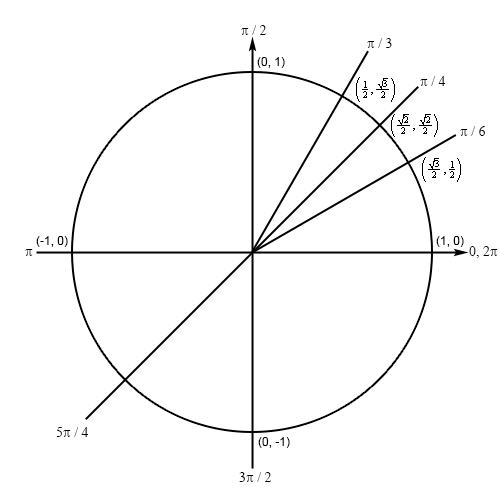Paul's Online Notes
Home / Calculus I / Review / Solving Trig Equations
Show Mobile Notice Show All Notes Hide All Notes
Mobile Notice
You appear to be on a device with a "narrow" screen width (i.e. you are probably on a mobile phone). Due to the nature of the mathematics on this site it is best views in landscape mode. If your device is not in landscape mode many of the equations will run off the side of your device (should be able to scroll to see them) and some of the menu items will be cut off due to the narrow screen width.

### Section 1.4 : Solving Trig Equations

7. Without using a calculator find the solution(s) to $$8\tan \left( {2x} \right) - 5 = 3$$ that are in $$\displaystyle \left[ { - \frac{\pi }{2},\frac{{3\pi }}{2}} \right]$$.

Show All Steps Hide All Steps

Hint : Find all the solutions to the equation without regard to the given interval. The first step in this process is to isolate the tangent (with a coefficient of one) on one side of the equation.
Start Solution

Isolating the tangent (with a coefficient of one) on one side of the equation gives,

$\tan \left( {2x} \right) = 1$
Hint : Determine all the angles in the range $$\left[ {0,2\pi } \right]$$ for which tangent will have this value.
Show Step 2

If tangent has a value of 1 then we know that sine and cosine must be the same. This means that, in the first quadrant, the solution is $$\frac{\pi }{4}$$. We also know that sine and cosine will be the same in the third quadrant and we can use the basic symmetry on our unit circle to determine this value. Here is a unit circle for this situation.By basic symmetry we can see that the line terminal line for the second angle must form an angle of $$\frac{\pi }{4}$$ with the negative $$x$$-axis as shown above and so it will be : $$\pi + \frac{\pi }{4} = \frac{{5\pi }}{4}$$.

Hint :Using the two angles above write down all the angles for which tangent will have this value and use these to write down all the solutions to the equation.
Show Step 3

From the discussion in the notes for this section we know that once we have these two angles we can get all possible angles by simply adding “$$+ \,2\pi n$$ for $$n = 0, \pm 1, \pm 2, \ldots$$” onto each of these.

This then means that we must have,

$2x = \frac{\pi }{4} + 2\pi n \hspace{0.5in} {\rm{OR }} \hspace{0.5in} 2x = \frac{{5\pi }}{4} + 2\pi n \hspace{0.5in} n = 0, \pm 1, \pm 2, \ldots$

Finally, to get all the solutions to the equation all we need to do is divide both sides by 2.

$x = \frac{\pi }{8} + \pi n \hspace{0.5in} {\rm{OR }} \hspace{0.5in} x = \frac{{5\pi }}{8} + \pi n \hspace{0.5in} n = 0, \pm 1, \pm 2, \ldots$
Hint : Now all we need to do is plug in values of $$n$$ to determine which solutions will actually fall in the given interval.
Show Step 4

Note that because at least some of the solutions will have a denominator of 8 it will probably be convenient to also have the interval written in terms of fractions with denominators of 8. Doing this will make it much easier to identify solutions that fall inside the interval so,

$\left[ { - \frac{\pi }{2},\frac{{3\pi }}{2}} \right] = \left[ { - \frac{{4\pi }}{8},\frac{{12\pi }}{8}} \right]$

With the interval written in this form, if our potential solutions have a denominator of 8, all we need to do is compare numerators. As long as the numerators are between $$- 4\pi$$ and $$12\pi$$ we’ll know that the solution is in the interval.

Also, in order to quickly determine the solution for particular values of $$n$$ it will be much easier to have both fractions in the solutions have denominators of 8. So, the solutions, written in this form, are.

$x = \frac{\pi }{8} + \frac{{8\pi n}}{8} \hspace{0.5in} {\rm{OR }} \hspace{0.5in} x = \frac{{5\pi }}{8} + \frac{{8\pi n}}{8} \hspace{0.5in} n = 0, \pm 1, \pm 2, \ldots$

Now let’s find all the solutions.

$\begin{array}{llcl}{n = - 1:} & {x = \require{cancel} \xcancel{{ - \frac{{7\pi }}{8}}} < - \frac{{4\pi }}{8}\,} & {\,\,\,{\rm{OR}}\,\,\,} & {x = - \frac{{3\pi }}{8}}\\ {n = 0:} & {x = \frac{\pi }{8}\,} & {\,\,\,{\rm{OR}}\,\,\,} & {x = \frac{{5\pi }}{8}}\\ {n = 1:} & {x = \frac{{9\pi }}{8}\,} & {\,\,\,{\rm{OR}}\,\,\,} & {x = \xcancel{{\frac{{13\pi }}{8}}} > \frac{{12\pi }}{8}}\end{array}$

There are a couple of things we should note before proceeding. First, it is important to understand both solutions from a given value of $$n$$ will not necessarily be in the given interval. It is completely possible, as this problem shows, that we will only get one or the other solution from a given value of $$n$$ to fall in the given interval.

Next notice that with each increase in $$n$$ we were really just adding/subtracting (depending upon the sign of $$n$$) another $$\frac{{8\pi }}{8}$$ from the previous results and by a quick inspection we could see that adding $$8\pi$$ to the numerator of the $$n = 1$$ solutions would result in numerators that are larger than $$12\pi$$ and so would result in solutions that are outside of the interval. Likewise, subtracting $$8\pi$$ from the $$n = - 1$$ solutions would result in numerators that are smaller than $$- 4\pi$$ and so would result in solutions that are outside the interval. Therefore, there is no reason to even go past the values of $$n$$ listed here.

So, it looks like we have the four solutions to this equation in the given interval.

$\require{bbox} \bbox[2pt,border:1px solid black]{{x = - \frac{{3\pi }}{8},\,\,\frac{\pi }{8},\,\,\frac{{5\pi }}{8},\,\,\frac{{9\pi }}{8}}}$